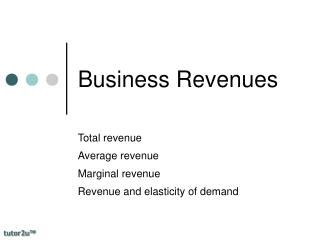DownloadDownload PresentationDownload Presentation- - - - - - - - - - - - - - - - - - - - - - - - - - - E N D - - - - - - - - - - - - - - - - - - - - - - - - - - -
##### Presentation Transcript

1. Business Revenues Total revenue Average revenue Marginal revenue Revenue and elasticity of demand

2. Revenue concepts • Revenue (or turnover) is the income generated from the sale of output in product markets. • There are two main revenue concepts to grasp at this stage: • Average Revenue (AR) = Price per unit = total revenue / output • Marginal Revenue (MR) = the change in revenue from selling one extra unit of output • Total revenue (TR) = price per unit x output

3. Numerical example

4. Numerical example

5. Marginal & Average Revenue Revenue P1 Average revenue P2 Q1 Q2 Output

6. Marginal & Average Revenue Revenue P1 Lost revenue from lower price Average revenue P2 Increased revenue from selling more Q1 Q2 Output

7. Marginal & Average Revenue Revenue P1 Average revenue P2 Marginal revenue Q1 Q2 Output

8. Maximising Total Revenue Total revenue maximised when MR = zero Revenue P1 AR Output Q1 MR

9. A Shift in Revenue Curves Revenue AR2 AR1 MR2 Output MR1

10. Importance of revenues • Revenues affect the profitability of a business • When demand is price inelastic, a fall in price causes a fall in TR (marginal revenue will be negative) • The elasticity of demand (AR) has an effect on the profit margins of businesses in competitive and concentrated markets • When demand is perfectly elastic, then the AR and the MR curves are the same